Ochem Incorporation-HOME

 Register Login
 Welcome to OChem Incorporation OChem Offers:   Advanced Chemicals, Custom Synthesis, Process Optimization and Scale-up Services
 Product Search Structural Search
 Shopping Cart: 0 item(s) Subtotal: \$0

FUNCTIONAL CATEGORIES

Total 93 Mycin products found.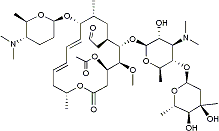Smiles: |CO[C@H]2[C@H](OC(C)=O)CC(=O)O[C@H](C)C/C=C/C=C/[C@H](O[C@H]1CC[C@H](N(C)C)[C@@H](C)O1)[C@H](C)C[C@H](CC=O)[C@@H]2O[C@@H]4O[C@H](C)[C@@H](O[C@H]3C[C@@](C)(O)[C@@H](O)[C@H](C)O3)[C@H](N(C)C)[C@H]4O|
 Catalog No.: 916A516 Product Name: Acetylspiramycin CAS Number: 24916-51-6 MDL Number: MFCD01684079 Formula: C45H76N2O15 FW: MSDS Pricing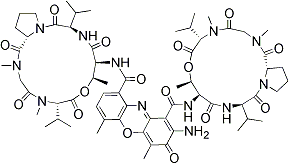Smiles: C[C@@H]1[C@@H](C(=O)N[C@@H](C(=O)N2CCC[C@H]2C(=O)N(CC(=O)N([C@H](C(=O)O1)C(C)C)C)C)C(C)C)NC(=O)C3=C4C(=C(C=C3)C)OC5=C(C(=O)C(=C(C5=N4)C(=O)N[C@H]6[C@H](OC(=O)[C@@H](N(C(=O)CN(C(=O)[C@@H]7CCCN7C(=O)[C@H](NC6=O)C(C)C)C)C)C(C)C)C)N)C
 Catalog No.: 50A760 Product Name: Actinomycin D CAS Number: 50-76-0 MDL Number: MFCD00005033 Formula: C62H86N12O16 FW: MSDS Pricing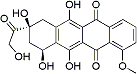Smiles: |COc3cccc4c(=O)c2c(O)c1C[C@@](O)(C(=O)CO)C[C@H](O)c1c(O)c2c(=O)c34|
 Catalog No.: 385A102 Product Name: Adriamycinone CAS Number: 24385-10-2 MDL Number: MFCD00871832 Formula: C21H18O9 FW: MSDS Pricing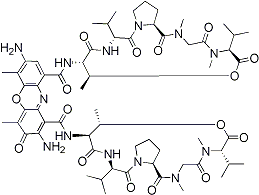Smiles: |Cc6c(N)cc(C(=O)N[C@@H]2C(=O)N[C@H](C(C)C)C(=O)N1CCC[C@H]1C(=O)N(C)CC(=O)N(C)[C@@H](C(C)C)C(=O)O[C@H]2C)c7nc5c(C(=O)N[C@@H]4C(=O)N[C@H](C(C)C)C(=O)N3CCC[C@H]3C(=O)N(C)CC(=O)N(C)[C@@H](C(C)C)C(=O)O[C@H]4C)c(N)c(=O)c(C)c5oc67|
 Catalog No.: 240A371 Product Name: 7-Aminoactinomycin D CAS Number: 7240-37-1 MDL Number: MFCD00077295 Formula: C62H87N13O16 FW: MSDS Pricing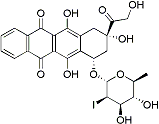Smiles: |C[C@@H]5O[C@@H](O[C@H]4C[C@](O)(C(=O)CO)Cc3c(O)c2c(=O)c1ccccc1c(=O)c2c(O)c34)[C@H](I)[C@H](O)[C@H]5O|
 Catalog No.: 689A491 Product Name: Annamycin CAS Number: 92689-49-1 MDL Number: MFCD00914599 Formula: C26H25IO11 FW: MSDS Pricing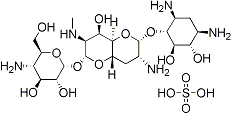Smiles: |O=S(=O)(O)O.CN[C@H]3[C@@H](O)[C@H]2O[C@H](O[C@@H]1[C@@H](N)C[C@@H](N)[C@H](O)[C@H]1O)[C@H](N)C[C@@H]2O[C@@H]3O[C@H]4O[C@H](CO)[C@@H](N)[C@H](O)[C@H]4O|
 Catalog No.: 710A078 Product Name: Apramycin sulfate CAS Number: 65710-07-8 MDL Number: MFCD06200257 Formula: C21H43N5O15S FW: MSDS Pricing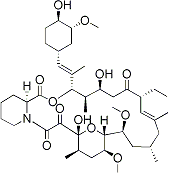Smiles: |CC[C@@H]3/C=C(C)C[C@H](C)C[C@H](OC)[C@H]4O[C@@](O)(C(=O)C(=O)N1CCCC[C@H]1C(=O)O[C@H](/C(C)=C[C@@H]2CC[C@@H](O)[C@H](OC)C2)[C@H](C)[C@@H](O)CC3=O)[C@H](C)C[C@@H]4OC|
 Catalog No.: 011A384 Product Name: Ascomycin CAS Number: 11011-38-4 MDL Number: MFCD06198665 Formula: C43H69NO12 FW: MSDS Pricing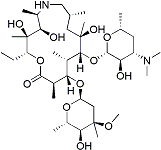Smiles: |CC[C@H]3OC(=O)[C@H](C)[C@@H](O[C@H]1C[C@@](C)(OC)[C@@H](O)[C@H](C)O1)C(C)[C@@H](O[C@@H]2O[C@H](C)C[C@H](N(C)C)[C@H]2O)[C@](C)(O)C[C@@H](C)CN[C@H](C)[C@@H](O)[C@]3(C)O|
 Catalog No.: 801A859 Product Name: Azathramycin CAS Number: 76801-85-9 MDL Number: MFCD09038719 Formula: C37H70N2O12 FW: MSDS Pricing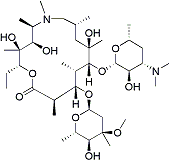Smiles: |CC[C@H]3OC(=O)[C@H](C)[C@@H](O[C@H]1C[C@@](C)(OC)[C@@H](O)[C@H](C)O1)C(C)[C@@H](O[C@@H]2O[C@H](C)C[C@H](N(C)C)[C@H]2O)[C@](C)(O)C[C@@H](C)CN(C)[C@H](C)[C@@H](O)[C@]3(C)O|
 Catalog No.: 905A015 Product Name: Azithromycin CAS Number: 83905-01-5 MDL Number: MFCD00873574 Formula: C38H72N2O12 FW: MSDS Pricing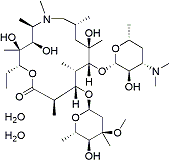Smiles: |O.O.CC[C@H]3OC(=O)[C@H](C)[C@@H](O[C@H]1C[C@@](C)(OC)[C@@H](O)[C@H](C)O1)C(C)[C@@H](O[C@@H]2O[C@H](C)C[C@H](N(C)C)[C@H]2O)[C@](C)(O)C[C@@H](C)CN(C)[C@H](C)[C@@H](O)[C@]3(C)O|
 Catalog No.: 772A700 Product Name: Azithromycin dihydrate CAS Number: 117772-70-0 MDL Number: MFCD01862248 Formula: C38H76N2O14 FW: MSDS Pricing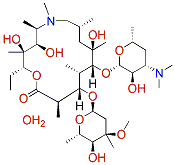Smiles: |O.CC[C@H]3OC(=O)[C@H](C)[C@@H](O[C@H]1C[C@@](C)(OC)[C@@H](O)[C@H](C)O1)C(C)[C@@H](O[C@@H]2O[C@H](C)C[C@H](N(C)C)[C@H]2O)[C@](C)(O)C[C@@H](C)CN(C)[C@H](C)[C@@H](O)[C@]3(C)O|
 Catalog No.: 470A244 Product Name: Azithromycin monohydrate CAS Number: 121470-24-4 MDL Number: MFCD11878626 Formula: C38H74N2O13 FW: MSDS Pricing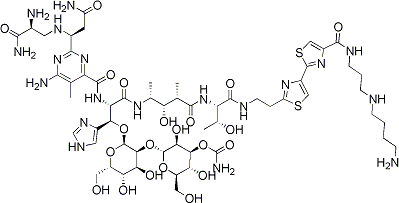Smiles: |Cc1c(N)nc([C@H](CC(N)=O)NC[C@H](N)C(N)=O)nc1C(=O)N[C@H](C(=O)N[C@H](C)[C@@H](O)[C@H](C)C(=O)N[C@H](C(=O)NCCc3nc(c2nc(C(=O)NCCCNCCCCN)cs2)cs3)[C@@H](C)O)[C@@H](O[C@@H]4O[C@@H](CO)[C@@H](O)[C@H](O)[C@@H]4O[C@H]5O[C@H](CO)[C@@H](O)[C@H](OC(N)=O)[C@@H]5O)c6c[nH]cn6|
 Catalog No.: 116B328 Product Name: Bleomycin a5 CAS Number: 11116-32-8 MDL Number: MFCD00869290 Formula: C57H89N19O21S2 FW: MSDS Pricing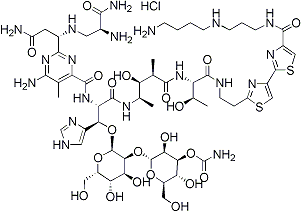Smiles: |Cl.Cc1c(N)nc([C@H](CC(N)=O)NC[C@H](N)C(N)=O)nc1C(=O)N[C@H](C(=O)N[C@H](C)[C@@H](O)[C@@H](C)C(=O)N[C@H](C(=O)NCCc3nc(c2nc(C(=O)NCCCNCCCCN)cs2)cs3)[C@@H](C)O)[C@@H](O[C@@H]4O[C@@H](CO)[C@@H](O)[C@H](O)[C@@H]4O[C@H]5O[C@H](CO)[C@@H](O)[C@H](OC(N)=O)[C@@H]5O)c6c[nH]cn6|
 Catalog No.: 658B474 Product Name: Bleomycin a5 hydrochloride CAS Number: 55658-47-4 MDL Number: MFCD01740724 Formula: C57H90ClN19O21S2 FW: MSDS PricingSmiles: CC1=C(N=C(N=C1N)[C@H](CC(=O)N)NC[C@@H](C(=O)N)N)C(=O)N[C@@H]([C@H](C2=CN=CN2)OC3C(C(C(C(O3)CO)O)O)OC4C(C(C(C(O4)CO)O)OC(=O)N)O)C(=O)N[C@H](C)[C@H]([C@H](C)C(=O)N[C@@H]([C@@H](C)O)C(=O)NCCC5=NC(=CS5)C6=NC(=CS6)C(=O)NCCC[S+](C)C)O.[Cl-]
 Catalog No.: 566B422 Product Name: Bleomycin chloride CAS Number: 49830-49-1 MDL Number: MFCD10566422 Formula: C55H85ClN17O21S3 FW: MSDS Pricing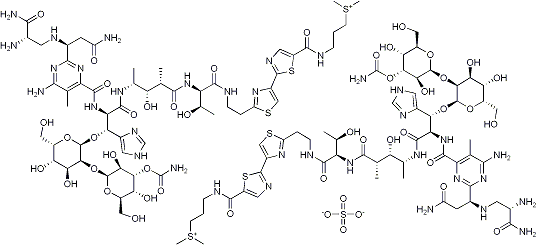Smiles: |O=S(=O)([O-])[O-].Cc1c(N)nc([C@H](CC(N)=O)NC[C@H](N)C(N)=O)nc1C(=O)N[C@@H](C(=O)N[C@H](C)[C@@H](O)[C@H](C)C(=O)N[C@@H](C(=O)NCCc3nc(c2ncc(C(=O)NCCC[S+](C)C)s2)cs3)[C@@H](C)O)[C@@H](O[C@@H]4O[C@@H](CO)[C@@H](O)[C@H](O)[C@@H]4O[C@@H]5O[C@H](CO)[C@@H](O)[C@@H](OC(N)=O)[C@@H]5O)c6c[nH]cn6.Cc1c(N)nc([C@H](CC(N)=O)NC[C@H](N)C(N)=O)nc1C(=O)N[C@@H](C(=O)N[C@H](C)[C@@H](O)[C@H](C)C(=O)N[C@@H](C(=O)NCCc3nc(c2ncc(C(=O)NCCC[S+](C)C)s2)cs3)[C@@H](C)O)[C@@H](O[C@@H]4O[C@@H](CO)[C@@H](O)[C@H](O)[C@@H]4O[C@@H]5O[C@H](CO)[C@@H](O)[C@@H](OC(N)=O)[C@@H]5O)c6c[nH]cn6|
 Catalog No.: 056B067 Product Name: Bleomycin sulfate CAS Number: 11056-06-7 MDL Number: MFCD00070310 Formula: C110H168N34O46S7 FW: MSDS Pricing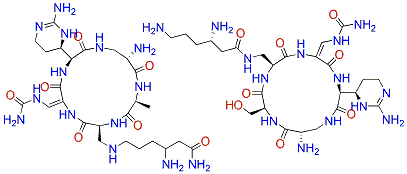Smiles: |C[C@@H]2NC(=O)[C@@H](N)CNC(=O)[C@H]([C@H]1CC/N=C(N)N1)NC(=O)/C(=CNC(N)=O)NC(=O)[C@H](CNCCCC(N)CC(N)=O)NC2=O.NCCC[C@H](N)CC(=O)NC[C@@H]2NC(=O)[C@H](CO)NC(=O)[C@@H](N)CNC(=O)[C@H]([C@H]1CC/N=C(N)N1)NC(=O)/C(=CNC(N)=O)NC2=O|
 Catalog No.: 003C386 Product Name: Capreomycin CAS Number: 11003-38-6 MDL Number: Formula: C50H88N28O15 FW: MSDS Pricing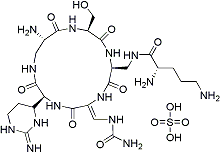Smiles: |O=S(=O)(O)O.N=C2NCCC([C@@H]1NC(=O)/C(=C/NC(N)=O)NC(=O)[C@H](CNC(=O)[C@@H](N)CCCN)NC(=O)[C@H](CO)NC(=O)[C@@H](N)CNC1=O)N2|
 Catalog No.: 405C374 Product Name: Capreomycin sulfate CAS Number: 1405-37-4 MDL Number: MFCD00079032 Formula: C24H44N14O12S FW: MSDS Pricing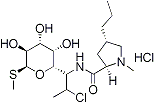Smiles: |Cl.CCC[C@H]2C[C@H](C(=O)NC(C(C)Cl)[C@@H]1O[C@@H](SC)[C@@H](O)[C@H](O)[C@@H]1O)N(C)C2|
 Catalog No.: 214C395 Product Name: (7S)-7-Chloro-7-deoxylincomycin hydrochloride CAS Number: 21462-39-5 MDL Number: MFCD09878730 Formula: C18H34Cl2N2O5S FW: MSDS Pricing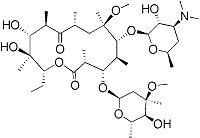Smiles: |CC[C@H]3OC(=O)[C@H](C)[C@@H](O[C@H]1C[C@@](C)(OC)[C@@H](O)[C@H](C)O1)[C@H](C)[C@@H](O[C@@H]2O[C@H](C)C[C@H](N(C)C)[C@H]2O)[C@](C)(OC)C[C@@H](C)C(=O)[C@H](C)[C@@H](O)[C@]3(C)O|
 Catalog No.: 103C119 Product Name: Clarithromycin CAS Number: 81103-11-9 MDL Number: MFCD00865140 Formula: C38H69NO13 FW: MSDS Pricing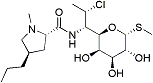Smiles: |CCC[C@@H]2C[C@@H](C(=O)N[C@H]([C@H](C)Cl)[C@H]1O[C@H](SC)[C@H](O)[C@@H](O)[C@H]1O)N(C)C2|
 Catalog No.: 323C449 Product Name: Clindamycin HCl salt CAS Number: 18323-44-9 MDL Number: MFCD00072005 Formula: C18H34Cl2N2O5S FW: MSDS PricingSmiles: |Cl.CCC[C@@H]2C[C@@H](C(=O)N[C@H]([C@H](C)Cl)[C@H]1O[C@H](SC)[C@H](O)[C@@H](O)[C@H]1O)N(C)C2|
 Catalog No.: 462C395 Product Name: Clindamycin hydrochloride CAS Number: 21462-39-5 MDL Number: MFCD07793327 Formula: C18H34Cl2N2O5S FW: MSDS Pricing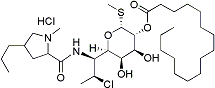Smiles: |Cl.CCCCCCCCCCCCCCCC(=O)O[C@@H]2[C@@H](O)[C@@H](O)[C@@H]([C@H](NC(=O)C1CC(CCC)CN1C)[C@H](C)Cl)O[C@@H]2SC|
 Catalog No.: 507C044 Product Name: Clindamycin palmitate HCl CAS Number: 25507-04-4 MDL Number: MFCD03093716 Formula: C34H64Cl2N2O6S FW: MSDS Pricing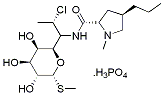Smiles: |O=P(O)(O)O.CCC[C@@H]2C[C@@H](C(=O)N[C@H]([C@H](C)Cl)[C@H]1O[C@H](SC)[C@H](O)[C@@H](O)[C@H]1O)N(C)C2|
 Catalog No.: 729C962 Product Name: Clindamycin phosphate CAS Number: 24729-96-2 MDL Number: MFCD07793328 Formula: C18H36ClN2O9PS FW: MSDS Pricing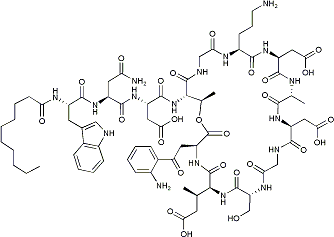Smiles: |CCCCCCCCCC(=O)N[C@@H](Cc1c[nH]c2ccccc12)C(=O)N[C@@H](CC(N)=O)C(=O)N[C@@H](CC(=O)O)C(=O)N[C@@H]4C(=O)NCC(=O)N[C@@H](CCCN)C(=O)N[C@@H](CC(=O)O)C(=O)N[C@H](C)C(=O)N[C@@H](CC(=O)O)C(=O)NCC(=O)N[C@H](CO)C(=O)N[C@@H]([C@H](C)CC(=O)O)C(=O)N[C@@H](CC(=O)c3ccccc3N)C(=O)O[C@@H]4C|
 Catalog No.: 060D533 Product Name: Daptomycin CAS Number: 103060-53-3 MDL Number: MFCD08282794 Formula: C72H101N17O26 FW: MSDS Pricing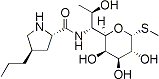Smiles: |CCC[C@H]2CN[C@H](C(=O)N[C@H]([C@@H](C)O)[C@H]1O[C@H](SC)[C@H](O)[C@@H](O)[C@H]1O)C2|
 Catalog No.: 256D168 Product Name: N-Demethyllincomycin CAS Number: 2256-16-8 MDL Number: MFCD13194821 Formula: C17H32N2O6S FW: MSDS Pricing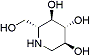Smiles: |OC[C@H]1NC[C@H](O)[C@@H](O)[C@@H]1O|
 Catalog No.: 30D962 Product Name: 1-Deoxynojirimycin CAS Number: 19130-96-2 MDL Number: MFCD00063474 Formula: C6H13NO4 FW: MSDS Pricing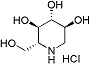Smiles: |Cl.OC[C@H]1NC[C@H](O)[C@@H](O)[C@@H]1O|
 Catalog No.: 732D504 Product Name: 1-Deoxynojirimycin hydrochloride CAS Number: 73285-50-4 MDL Number: MFCD00133247 Formula: C6H14ClNO4 FW: MSDS Pricing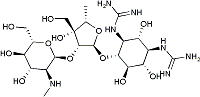Smiles: |CN[C@H]1[C@H](O)[C@@H](O)[C@H](CO)O[C@H]1O[C@H]3[C@H](O[C@H]2[C@H](O)[C@@H](O)[C@H](NC(=N)N)[C@@H](O)[C@@H]2NC(=N)N)O[C@@H](C)[C@]3(O)CO|
 Catalog No.: 128D461 Product Name: Dihydrostreptomycin CAS Number: 128-46-1 MDL Number: MFCD00084778 Formula: C21H41N7O12 FW: MSDS Pricing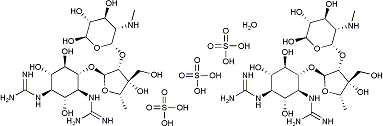Smiles: |O.O=S(=O)(O)O.O=S(=O)(O)O.O=S(=O)(O)O.CN[C@H]1[C@H](O)[C@@H](O)[C@H](O)O[C@H]1O[C@H]3[C@H](O[C@H]2[C@H](O)[C@@H](O)[C@H](NC(=N)N)[C@@H](O)[C@@H]2NC(=N)N)O[C@@H](C)[C@]3(O)CO.CN[C@H]1[C@H](O)[C@@H](O)[C@H](O)O[C@H]1O[C@H]3[C@H](O[C@H]2[C@H](O)[C@@H](O)[C@H](NC(=N)N)[C@@H](O)[C@@H]2NC(=N)N)O[C@@H](C)[C@]3(O)CO|
 Catalog No.: 90D277 Product Name: Dihydrostreptomycin sesquisulfate hydrate CAS Number: 5490-27-7 MDL Number: MFCD00167162 Formula: C40H86N14O37S3 FW: MSDS Pricing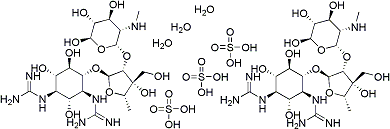Smiles: |O.O.O.O=S(=O)(O)O.O=S(=O)(O)O.O=S(=O)(O)O.CN[C@H]1[C@H](O)[C@@H](O)[C@H](O)O[C@H]1O[C@H]3[C@H](O[C@H]2[C@H](O)[C@@H](O)[C@H](NC(=N)N)[C@@H](O)[C@@H]2NC(=N)N)O[C@@H](C)[C@]3(O)CO.CN[C@H]1[C@H](O)[C@@H](O)[C@H](O)O[C@H]1O[C@H]3[C@H](O[C@H]2[C@H](O)[C@@H](O)[C@H](NC(=N)N)[C@@H](O)[C@@H]2NC(=N)N)O[C@@H](C)[C@]3(O)CO|
 Catalog No.: 490D277 Product Name: Dihydrostreptomycin sesquisulfate trihydrate CAS Number: MDL Number: MFCD08457669 Formula: C40H90N14O39S3 FW: MSDS Pricing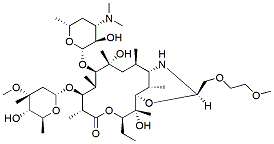Smiles: |CC[C@H]4OC(=O)[C@H](C)[C@@H](O[C@H]1C[C@@](C)(OC)[C@@H](O)[C@H](C)O1)[C@H](C)[C@@H](O[C@@H]2O[C@H](C)C[C@H](N(C)C)[C@H]2O)[C@](C)(O)C[C@@H](C)[C@@H]3N[C@@H](COCCOC)O[C@H]([C@H]3C)[C@]4(C)O|
 Catalog No.: 013D041 Product Name: Dirithromycin CAS Number: 62013-04-1 MDL Number: MFCD00865041 Formula: C42H78N2O14 FW: MSDS Pricing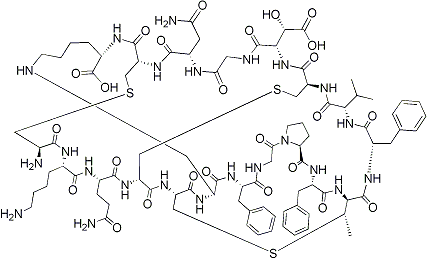Smiles: |CC(C)[C@@H]5NC(=O)[C@H](Cc1ccccc1)NC(=O)[C@@H]7NC(=O)[C@H](Cc2ccccc2)NC(=O)[C@@H]3CCCN3C(=O)CNC(=O)[C@H](Cc4ccccc4)NC(=O)[C@H]8CNCCCC[C@@H](C(=O)O)NC(=O)[C@@H]6CSC[C@H](N)C(=O)N[C@@H](CCCCN)C(=O)N[C@@H](CCC(N)=O)C(=O)N[C@@H](CSC[C@H](NC5=O)C(=O)N[C@@H]([C@H](O)C(=O)O)C(=O)NCC(=O)N[C@@H](CC(N)=O)C(=O)N6)C(=O)N[C@H](CS[C@H]7C)C(=O)N8|
 Catalog No.: 391D362 Product Name: Duramycin CAS Number: 1391-36-2 MDL Number: MFCD00133301 Formula: C88H123N23O25S3 FW: MSDS Pricing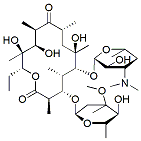Smiles: |CC[C@H]3OC(=O)[C@H](C)[C@@H](O[C@H]1C[C@@](C)(OC)[C@@H](O)[C@H](C)O1)[C@H](C)[C@@H](O[C@@H]2O[C@H](C)C[C@H](N(C)C)[C@H]2O)[C@](C)(O)C[C@@H](C)C(=O)[C@H](C)[C@@H](O)[C@]3(C)O|
 Catalog No.: 114E078 Product Name: Erythromycin CAS Number: 114-07-8 MDL Number: MFCD00084654 Formula: C37H67NO13 FW: MSDS Pricing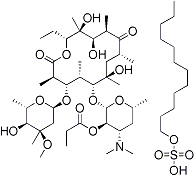Smiles: |CCCCCCCCCCCCOS(=O)(=O)O.CCC(=O)O[C@H]3[C@H](O[C@@H]2[C@@H](C)[C@H](O[C@H]1C[C@@](C)(OC)[C@@H](O)[C@H](C)O1)[C@@H](C)C(=O)O[C@H](CC)[C@@](C)(O)[C@H](O)[C@@H](C)C(=O)[C@H](C)C[C@@]2(C)O)O[C@H](C)C[C@@H]3N(C)C|
 Catalog No.: 521E628 Product Name: Erythromycin estolate CAS Number: 3521-62-8 MDL Number: MFCD00084691 Formula: C52H97NO18S FW: MSDS Pricing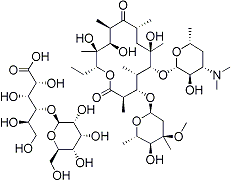Smiles: |O=C(O)[C@H](O)[C@@H](O)[C@H](O[C@@H]1O[C@H](CO)[C@@H](O)[C@@H](O)[C@H]1O)[C@H](O)CO.CC[C@H]3OC(=O)[C@H](C)[C@@H](O[C@H]1C[C@@](C)(OC)[C@@H](O)[C@H](C)O1)[C@H](C)[C@@H](O[C@@H]2O[C@H](C)C[C@H](N(C)C)[C@H]2O)[C@](C)(O)C[C@@H](C)C(=O)[C@H](C)[C@@H](O)[C@]3(C)O|
 Catalog No.: 847E298 Product Name: Erythromycin lactobionate CAS Number: 3847-29-8 MDL Number: MFCD01716346 Formula: C49H89NO25 FW: MSDS Pricing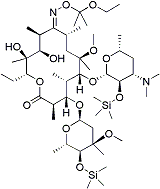Smiles: |CCOC(C)(C)O/N=C/3[C@H](C)C[C@@](C)(OC)[C@H](O[C@@H]1O[C@H](C)C[C@H](N(C)C)[C@H]1O[Si](C)(C)C)C(C)[C@H](O[C@H]2C[C@@](C)(OC)[C@@H](O[Si](C)(C)C)[C@H](C)O2)[C@@H](C)C(=O)O[C@H](CC)[C@@](C)(O)[C@H](O)[C@H]3C|
 Catalog No.: 665E622 Product Name: Erythromycin,6-O-methyl-2',4''-bis-O-(trimethylsilyl)-,9-[o-(1-ethoxy-1-methylethyl)oxime] CAS Number: 119665-62-2 MDL Number: MFCD09263749 Formula: C49H96N2O14Si2 FW: MSDS Pricing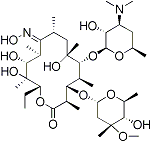Smiles: |CC[C@H]3OC(=O)[C@H](C)[C@@H](O[C@H]1C[C@@](C)(OC)[C@@H](O)[C@H](C)O1)[C@H](C)[C@@H](O[C@@H]2O[C@H](C)C[C@H](N(C)C)[C@H]2O)[C@](C)(O)C[C@@H](C)/C(=N/O)[C@H](C)[C@@H](O)[C@]3(C)O|
 Catalog No.: 127E189 Product Name: Erythromycin-9-oxime CAS Number: 13127-18-9 MDL Number: MFCD00914803 Formula: C37H68N2O13 FW: MSDS Pricing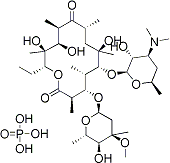Smiles: |O=P(O)(O)O.CC[C@H]3OC(=O)[C@H](C)[C@@H](O[C@H]1C[C@@](C)(OC)[C@@H](O)[C@H](C)O1)[C@H](C)[C@@H](O[C@@H]2O[C@H](C)C[C@H](N(C)C)[C@H]2O)[C@](C)(O)C[C@@H](C)C(=O)[C@H](C)[C@@H](O)[C@]3(C)O|
 Catalog No.: 501E002 Product Name: Erythromycin phosphate CAS Number: 4501-00-2 MDL Number: MFCD00270635 Formula: C37H70NO17P FW: MSDS Pricing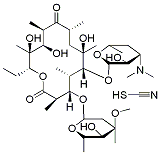Smiles: |N#CS.CC[C@H]3OC(=O)[C@H](C)[C@@H](O[C@H]1C[C@@](C)(OC)[C@@H](O)[C@H](C)O1)C(C)[C@@H](O[C@@H]2O[C@H](C)C[C@H](N(C)C)[C@H]2O)[C@](C)(O)C[C@@H](C)C(=O)[C@H](C)[C@@H](O)[C@]3(C)O|
 Catalog No.: 704E678 Product Name: Erythromycin thiocyanate CAS Number: 7704-67-8 MDL Number: MFCD00072026 Formula: C38H68N2O13S FW: MSDS Pricing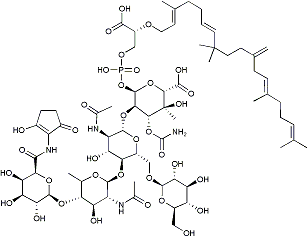Smiles: |C=C(C/C=C(C)/CC/C=C(C)C)CCC(C)(C)/C=C/CC/C(C)=C/CO[C@H](COP(=O)(O)O[C@H]1O[C@H](C(=O)O)[C@@](C)(O)[C@H](OC(N)=O)[C@H]1O[C@@H]6O[C@H](CO[C@@H]2O[C@H](CO)[C@@H](O)[C@H](O)[C@H]2O)[C@@H](O[C@@H]5OC(C)[C@@H](O[C@@H]4O[C@H](C(=O)N/C3=C(O)/CCC3=O)[C@H](O)[C@H](O)[C@H]4O)[C@H](O)[C@H]5NC(C)=O)[C@H](O)[C@H]6NC(C)=O)C(=O)O|
 Catalog No.: 015F375 Product Name: Flavomycin CAS Number: 11015-37-5 MDL Number: MFCD01742468 Formula: C69H107N4O35P FW: MSDS Pricing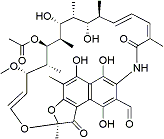Smiles: |CO[C@H]1/C=C/O[C@@]4(C)Oc3c(C)c(O)c2c(O)c(NC(=O)/C(C)=CC=C[C@H](C)[C@H](O)[C@@H](C)[C@@H](O)[C@@H](C)[C@H](OC(C)=O)[C@@H]1C)c(C=O)c(O)c2c3C4=O|
 Catalog No.: 292F223 Product Name: 3-Formyl rifamycin SV CAS Number: 13292-22-3 MDL Number: MFCD01729454 Formula: C38H47NO13 FW: MSDS Pricing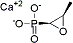Smiles: |[Ca+2].C[C@H]1O[C@H]1P(=O)([O-])[O-]|
 Catalog No.: 016F988 Product Name: Fosfomycin calcium salt CAS Number: 26016-98-8 MDL Number: MFCD00070095 Formula: C3H5CaO4P FW: MSDS Pricing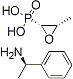Smiles: |C[C@@H]1O[C@@H]1P(=O)(O)O.C[C@@H](N)c1ccccc1|
 Catalog No.: 383F077 Product Name: Fosfomycin phenylethylamine CAS Number: 25383-07-7 MDL Number: MFCD09839038 Formula: C11H18NO4P FW: MSDS Pricing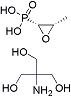Smiles: |C[C@@H]1O[C@@H]1P(=O)(O)O.NC(CO)(CO)CO|
 Catalog No.: 964F859 Product Name: Fosfomycin trometamol CAS Number: 78964-85-9 MDL Number: MFCD01706261 Formula: C7H18NO7P FW: MSDS Pricing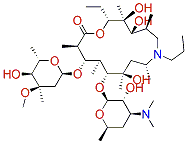Smiles: |CCCN3C[C@H](C)[C@@H](O)[C@](C)(O)[C@@H](CC)OC(=O)[C@H](C)[C@@H](O[C@H]1C[C@@](C)(OC)[C@@H](O)[C@H](C)O1)[C@H](C)[C@@H](O[C@@H]2O[C@H](C)C[C@H](N(C)C)[C@H]2O)[C@](C)(O)C[C@H]3C|
 Catalog No.: 435G729 Product Name: Gamithromycin CAS Number: 145435-72-9 MDL Number: MFCD09954125 Formula: C40H76N2O12 FW: MSDS Pricing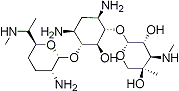Smiles: |CNC(C)[C@@H]3CC[C@@H](N)[C@@H](O[C@@H]2[C@@H](N)C[C@@H](N)[C@H](O[C@H]1OC[C@](C)(O)[C@H](NC)[C@H]1O)[C@H]2O)O3|
 Catalog No.: 403G663 Product Name: Gentamycin CAS Number: 1403-66-3 MDL Number: MFCD00864946 Formula: C21H43N5O7 FW: MSDS Pricing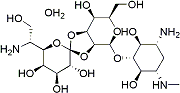Smiles: |CN[C@H]4C[C@@H](N)[C@H](O)[C@@H](O[C@@H]2O[C@H](CO)[C@H](O)[C@@H]3O[C@]1(O[C@H]([C@H](N)CO)[C@H](O)[C@H](O)[C@H]1O)O[C@H]23)[C@@H]4O|
 Catalog No.: 82H049 Product Name: Hygromycin B CAS Number: 31282-04-9 MDL Number: MFCD06795479 Formula: C20H37N3O13 FW: MSDS PricingSmiles: |O.CN[C@H]4C[C@@H](N)[C@H](O)[C@@H](O[C@@H]2O[C@H](CO)[C@H](O)[C@@H]3O[C@]1(O[C@H]([C@H](N)CO)[C@H](O)[C@H](O)[C@H]1O)O[C@H]23)[C@@H]4O|
 Catalog No.: 282H049 Product Name: HygromycinBhydrate CAS Number: 31282-04-9 MDL Number: MFCD06795480 Formula: C20H39N3O14 FW: MSDS Pricing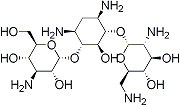Smiles: |NC[C@H]3O[C@H](O[C@H]2[C@H](N)C[C@H](N)[C@@H](O[C@H]1O[C@H](CO)[C@@H](O)[C@H](N)[C@H]1O)[C@@H]2O)[C@H](N)[C@@H](O)[C@@H]3O|
 Catalog No.: 696K768 Product Name: Kanamycin b CAS Number: 4696-76-8 MDL Number: MFCD01683541 Formula: C18H37N5O10 FW: MSDS Pricing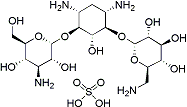Smiles: |O=S(=O)(O)O.NC[C@H]3O[C@H](O[C@@H]2[C@@H](N)C[C@@H](N)[C@H](O[C@H]1O[C@H](CO)[C@@H](O)[C@H](N)[C@H]1O)[C@H]2O)[C@H](O)[C@@H](O)[C@@H]3O|
 Catalog No.: 133K926 Product Name: Kanamycin sulfate CAS Number: 133-92-6 MDL Number: MFCD00070253 Formula: C18H38N4O15S FW: MSDS PricingSmiles: |Cl.C[C@H]2O[C@H](O[C@H]1[C@H](O)[C@@H](O)[C@@H](O)[C@H](O)[C@@H]1O)[C@@H](N)C[C@@H]2NC(=N)C(=O)O|
 Catalog No.: 980K183 Product Name: Kasugamycin hydrochloride CAS Number: 6980-18-3 MDL Number: MFCD00058459 Formula: C14H26ClN3O9 FW: MSDS Pricing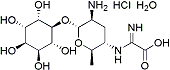Smiles: |O.Cl.C[C@H]2O[C@H](O[C@H]1[C@H](O)[C@@H](O)[C@@H](O)[C@H](O)[C@@H]1O)[C@@H](N)C[C@@H]2NC(=N)C(=O)O|
 Catalog No.: 408K469 Product Name: Kasugamycin hydrochloride hydrate CAS Number: 19408-46-9 MDL Number: MFCD00149375 Formula: C14H28ClN3O10 FW: MSDS Pricing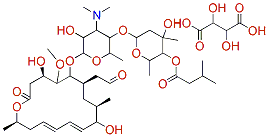Smiles: |O=C(O)C(O)C(O)C(=O)O.COC1[C@H](O)CC(=O)O[C@H](C)C/C=C/C=C/C(O)[C@H](C)C[C@H](CC=O)C1OC3OC(C)C(OC2CC(C)(O)C(OC(=O)CC(C)C)C(C)O2)C(N(C)C)C3O|
 Catalog No.: 280K561 Product Name: Kitasamycin tartrate CAS Number: 37280-56-1 MDL Number: Formula: C44H73NO20 FW: MSDS Pricing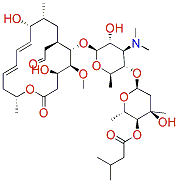Smiles: |CO[C@H]1[C@H](O)CC(=O)O[C@H](C)C/C=C/C=C/[C@H](O)[C@H](C)C[C@H](CC=O)[C@@H]1O[C@@H]3O[C@H](C)[C@@H](O[C@H]2C[C@@](C)(O)[C@@H](OC(=O)CC(C)C)[C@H](C)O2)[C@H](N(C)C)[C@H]3O|
 Catalog No.: 846L347 Product Name: Leucomycin A1 CAS Number: 16846-34-7 MDL Number: MFCD00865001 Formula: C40H67NO14 FW: MSDS Pricing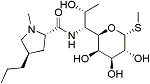Smiles: |CCC[C@@H]2C[C@@H](C(=O)N[C@H]([C@@H](C)O)[C@H]1O[C@H](SC)[C@H](O)[C@@H](O)[C@H]1O)N(C)C2|
 Catalog No.: 54L212 Product Name: Lincomycin CAS Number: 154-21-2 MDL Number: MFCD00135816 Formula: C18H34N2O6S FW: MSDS Pricing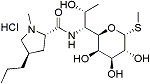Smiles: |Cl.CCC[C@@H]2C[C@@H](C(=O)N[C@H]([C@@H](C)O)[C@H]1O[C@H](SC)[C@H](O)[C@@H](O)[C@H]1O)N(C)C2|
 Catalog No.: 859L187 Product Name: Lincomycin hydrochloride CAS Number: 859-18-7 MDL Number: MFCD00083647 Formula: C18H35ClN2O6S FW: MSDS Pricing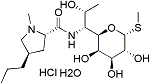Smiles: |O.Cl.CCC[C@@H]2C[C@@H](C(=O)N[C@H]([C@@H](C)O)[C@H]1O[C@H](SC)[C@H](O)[C@@H](O)[C@H]1O)N(C)C2|
 Catalog No.: 179L499 Product Name: Lincomycin hydrochloride monohydrate CAS Number: 7179-49-9 MDL Number: MFCD00167245 Formula: C18H37ClN2O7S FW: MSDS Pricing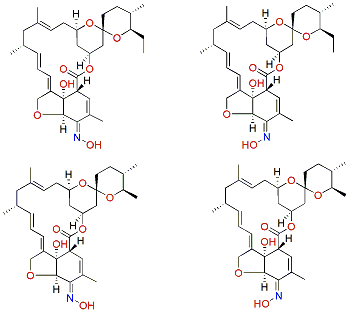Smiles: |CC[C@H]1O[C@]5(CC[C@@H]1C)C[C@@H]2C[C@@H](C/C=C(C)/C[C@@H](C)/C=C/C=C/3CO[C@@H]4/C(=N/O)/C(C)=CC(C(=O)O2)C34O)O5.C/C3=CC[C@H]2C[C@@H](C[C@]1(CC[C@H](C)[C@@H](C)O1)O2)OC(=O)[C@H]4/C=C(C)C(=NO)[C@H]5OC/C(=C/C=C/[C@H](C)C3)[C@@]45O.C/C3=CC[C@H]2C[C@@H](C[C@]1(CC[C@H](C)[C@@H](C)O1)O2)OC(=O)[C@H]4/C=C(C)C(=N/O)[C@H]5OC/C(=C/C=C/[C@H](C)C3)[C@@]45O.CC[C@H]1O[C@]5(CC[C@@H]1C)C[C@@H]2C[C@H](C/C=C(C)/C[C@@H](C)/C=C/C=C/3CO[C@@H]4/C(=NO)/C(C)=C[C@H](C(=O)O2)[C@]34O)O5|
 Catalog No.: 496M102 Product Name: Milbemycin oxime CAS Number: 129496-10-2 MDL Number: MFCD09038910 Formula: C126H176N4O28 FW: MSDS Pricing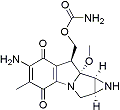Smiles: |CO[C@@]24[C@H](COC(N)=O)c1c(c(=O)c(C)c(N)c1=O)N2C[C@@H]3N[C@@H]34|
 Catalog No.: 078M109 Product Name: Mitomycin C CAS Number: 50-07-7 MDL Number: MFCD00078109 Formula: C15H18N4O5 FW: MSDS Pricing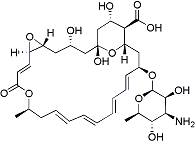Smiles: |C[C@@H]3C/C=C/C=C/C=C/C=C/[C@H](O[C@@H]1O[C@H](C)[C@@H](O)[C@H](N)[C@@H]1O)C[C@@H]4O[C@](O)(C[C@@H](O)C[C@H]2O[C@H]2/C=C/C(=O)O3)C[C@H](O)[C@H]4C(=O)O|
 Catalog No.: 681N938 Product Name: Natamycin CAS Number: 7681-93-8 MDL Number: MFCD00135085 Formula: C33H47NO13 FW: MSDS Pricing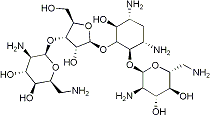Smiles: |NC[C@H]4O[C@H](O[C@@H]1[C@@H](N)C[C@@H](N)[C@H](O)C1O[C@@H]3O[C@H](CO)[C@@H](O[C@H]2O[C@@H](CN)[C@@H](O)[C@H](O)[C@H]2N)[C@H]3O)[C@H](N)[C@@H](O)[C@@H]4O|
 Catalog No.: 119N040 Product Name: Neomycin B CAS Number: 119-04-0 MDL Number: MFCD00242602 Formula: C23H46N6O13 FW: MSDS PricingSmiles: |O=S(=O)(O)O.NC[C@H]4O[C@H](O[C@@H]1[C@@H](N)C[C@@H](N)[C@H](O)[C@H]1O[C@@H]3O[C@H](CO)[C@@H](O[C@H]2O[C@@H](CN)[C@@H](O)[C@H](O)[C@H]2N)[C@H]3O)[C@H](N)[C@@H](O)[C@@H]4O|
 Catalog No.: 405N103 Product Name: Neomycin sulfate CAS Number: 1405-10-3 MDL Number: MFCD00037007 Formula: C23H48N6O17S FW: MSDS Pricing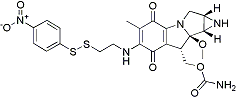Smiles: |CO[C@@]35[C@H](COC(N)=O)c2c(c(=O)c(C)c(NCCSSc1ccc(N(=O)=O)cc1)c2=O)N3C[C@@H]4N[C@@H]45|
 Catalog No.: 056N363 Product Name: N-7-(2-(Nitrophenyldithio)ethyl)mitomycin c CAS Number: 95056-36-3 MDL Number: MFCD13194737 Formula: C23H25N5O7S2 FW: MSDS Pricing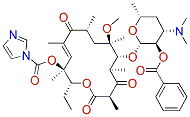Smiles: |CC[C@H]3OC(=O)[C@H](C)C(=O)[C@H](C)[C@@H](O[C@@H]1O[C@H](C)C[C@H](N(C)C)[C@H]1OC(=O)c2ccccc2)[C@](C)(OC)C[C@@H](C)C(=O)/C(C)=C/[C@]3(C)OC(=O)n4ccnc4|
 Catalog No.: 145O835 Product Name: 2 '-Obenzoyl-3-O-de [(2,6-dideoxy-3-c-methyl-3-O-methyl-a-L-ribohexopyranosyl) oxy]-11-deoxy-10,11-didehydro-12-O-[(1H-imidazol-1-yl) carbonyl]-6-O-methyl-3-oxo erythromycin CAS Number: 160145-83-5 MDL Number: MFCD09750997 Formula: C41H57N3O11 FW: MSDS Pricing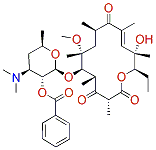Smiles: |CC[C@H]3OC(=O)[C@H](C)C(=O)[C@H](C)[C@@H](O[C@@H]1O[C@H](C)C[C@H](N(C)C)[C@H]1OC(=O)c2ccccc2)[C@](C)(OC)C[C@@H](C)C(=O)/C(C)=C/[C@]3(C)O|
 Catalog No.: 145O824 Product Name: 2 '-Obenzoyl-3-O-de [(2,6-dideoxy-3-c-methyl-3-O-methyl-a-L-ribohexopyranosyl)oxy]-11-deoxy-10,11-didehydro-6-O-methyl-3-oxo erythromycin CAS Number: 160145-82-4 MDL Number: MFCD09751204 Formula: C37H55NO10 FW: MSDS Pricing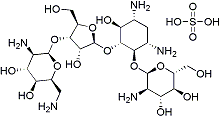Smiles: |O=S(=O)(O)O.NC[C@@H]4O[C@H](O[C@H]3[C@@H](O)[C@H](O[C@@H]1[C@@H](O)[C@H](N)C[C@H](N)[C@H]1O[C@H]2O[C@H](CO)[C@@H](O)[C@H](O)[C@H]2N)O[C@@H]3CO)[C@H](N)[C@@H](O)[C@@H]4O|
 Catalog No.: 263P894 Product Name: Paromomycin sulfate CAS Number: 1263-89-4 MDL Number: MFCD00079278 Formula: C23H47N5O18S FW: MSDS Pricing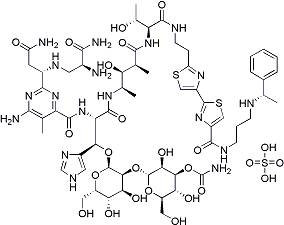Smiles: |O=S(=O)(O)O.Cc1c(N)nc([C@H](CC(N)=O)NC[C@H](N)C(N)=O)nc1C(=O)N[C@H](C(=O)N[C@H](C)[C@@H](O)[C@H](C)C(=O)N[C@H](C(=O)NCCc4nc(c3nc(C(=O)NCCCN[C@@H](C)c2ccccc2)cs3)cs4)[C@@H](C)O)[C@@H](O[C@@H]5O[C@@H](CO)[C@@H](O)[C@H](O)[C@@H]5O[C@H]6O[C@H](CO)[C@@H](O)[C@H](OC(N)=O)[C@@H]6O)c7c[nH]cn7|
 Catalog No.: 384P291 Product Name: Peplomycin sulfate CAS Number: 70384-29-1 MDL Number: MFCD01708900 Formula: C61H90N18O25S3 FW: MSDS Pricing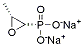Smiles: |[Na+].[Na+].C[C@H]1O[C@H]1P(=O)([O-])[O-]|
 Catalog No.: 016P999 Product Name: Phosphomycin disodium salt CAS Number: 26016-99-9 MDL Number: MFCD00056853 Formula: C3H5Na2O4P FW: MSDS Pricing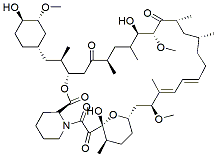Smiles: |CO[C@H]4C[C@@H]1CC[C@@H](C)[C@@](O)(O1)C(=O)C(=O)N2CCCC[C@H]2C(=O)O[C@H]([C@H](C)C[C@@H]3CC[C@@H](O)[C@H](OC)C3)CC(=O)[C@H](C)/C=C(C)[C@@H](O)[C@@H](OC)C(=O)[C@H](C)C[C@H](C)/C=CC=C/C=C4/C|
 Catalog No.: 123R889 Product Name: Rapamycin CAS Number: 53123-88-9 MDL Number: MFCD00867594 Formula: C51H79NO13 FW: MSDS Pricing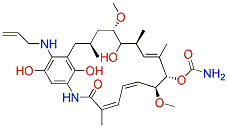Smiles: |C=CCNc1c(O)cc2NC(=O)/C(C)=CC=C/[C@H](OC)[C@@H](OC(N)=O)/C(C)=C[C@H](C)C(O)[C@@H](OC)C[C@@H](C)Cc1c2O|
 Catalog No.: 402R234 Product Name: Retaspimycin CAS Number: 857402-23-4 MDL Number: Formula: C31H45N3O8 FW: MSDS Pricing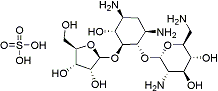Smiles: |O=S(=O)(O)O.NC[C@@H]3O[C@@H](O[C@H]1[C@H](N)C[C@H](N)[C@@H](O)[C@@H]1O[C@@H]2O[C@H](CO)[C@@H](O)[C@H]2O)[C@@H](N)[C@H](O)[C@H]3O|
 Catalog No.: 546R650 Product Name: Ribostamycin sulfate CAS Number: 25546-65-0 MDL Number: MFCD00057496 Formula: C17H36N4O14S FW: MSDS Pricing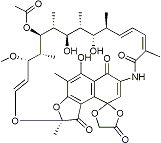Smiles: |CO[C@H]5/C=C/O[C@@]4(C)Oc3c(C)c(O)c2C(=O)/C(=CC1(OCC(=O)O1)c2c3C4=O)NC(=O)/C(C)=CC=C[C@H](C)[C@H](O)[C@@H](C)[C@@H](O)[C@@H](C)[C@H](OC(C)=O)[C@@H]5C|
 Catalog No.: 487R059 Product Name: Rifamycin O CAS Number: 14487-05-9 MDL Number: MFCD01683928 Formula: C39H47NO14 FW: MSDS Pricing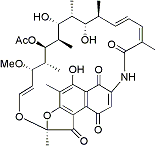Smiles: |CO[C@H]4/C=C/O[C@@]3(C)Oc2c(C)c(O)c1c(=O)c(cc(=O)c1c2C3=O)NC(=O)/C(C)=CC=C[C@H](C)[C@H](O)[C@@H](C)[C@@H](O)[C@@H](C)[C@H](OC(C)=O)[C@@H]4C|
 Catalog No.: 553R792 Product Name: Rifamycin S CAS Number: 13553-79-2 MDL Number: MFCD06198807 Formula: C37H45NO12 FW: MSDS PricingSmiles: |[Na+].COC(=O)[C@@H]1[C@@H](C)[C@@H](O)[C@H](C)[C@H](O)[C@H](C)/C=C/C=C(C)C(=O)Nc4cc([O-])c3c2C(=O)[C@@](C)(O/C=C/[C@H](OC)[C@@H]1C)Oc2c(C)c(O)c3c4O|
 Catalog No.: 897R393 Product Name: Rifamycin sodium salt CAS Number: 14897-39-3 MDL Number: MFCD00056847 Formula: C37H46NNaO12 FW: MSDS Pricing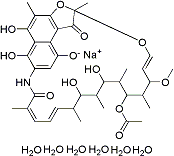Smiles: |O.O.O.O.O.O.[Na+].COC4/C=C/OC3(C)Oc2c(C)c(O)c1c(O)c(cc([O-])c1c2C3=O)NC(=O)/C(C)=CC=CC(C)C(O)C(C)C(O)C(C)C(OC(C)=O)C4C|
 Catalog No.: W97R393 Product Name: Rifamycin SV sodium salt hexahydrate CAS Number: MDL Number: MFCD00167105 Formula: C37H58NNaO18 FW: MSDS Pricing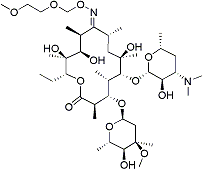Smiles: |CC[C@H]3OC(=O)[C@H](C)[C@@H](O[C@H]1C[C@@](C)(OC)[C@@H](O)[C@H](C)O1)[C@H](C)[C@@H](O[C@@H]2O[C@H](C)C[C@H](N(C)C)[C@H]2O)[C@](C)(O)C[C@@H](C)/C(=N/OCOCCOC)[C@H](C)[C@@H](O)[C@]3(C)O|
 Catalog No.: 214R831 Product Name: Roxithromycin CAS Number: 80214-83-1 MDL Number: MFCD00214389 Formula: C41H76N2O15 FW: MSDS Pricing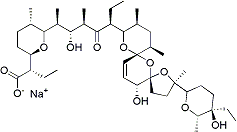Smiles: |CC[C@H](C(=O)O)[C@H]5CC[C@H](C)[C@H]([C@@H](C)[C@H](O)[C@H](C)C(=O)[C@H](CC)[C@H]4O[C@]3(/C=C[C@@H](O)[C@]2(CC[C@@](C)([C@H]1CC[C@](O)(CC)[C@H](C)O1)O2)O3)[C@H](C)C[C@@H]4C)O5|
 Catalog No.: 003S104 Product Name: Salinomycin CAS Number: 53003-10-4 MDL Number: MFCD00865281 Formula: C42H70O11 FW: MSDS PricingSmiles: |[Na+].CC[C@H](C(=O)[O-])[C@H]5CC[C@H](C)C([C@@H](C)[C@@H](O)[C@@H](C)C(=O)[C@@H](CC)C4O[C@]3(/C=C[C@@H](O)[C@]2(CC[C@](C)(C1CC[C@](O)(CC)[C@H](C)O1)O2)O3)[C@H](C)C[C@@H]4C)O5|
 Catalog No.: 721S318 Product Name: Salinomycin sodium CAS Number: 55721-31-8 MDL Number: MFCD00036900 Formula: C42H69NaO11 FW: MSDS Pricing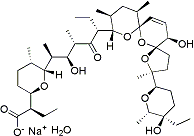Smiles: |O.[Na+].CC[C@@H](C(=O)[O-])[C@H]5CC[C@H](C)[C@H]([C@@H](C)[C@H](O)[C@H](C)C(=O)[C@H](CC)[C@H]4O[C@]3(/C=C[C@@H](O)[C@]2(CC[C@@](C)([C@H]1CC[C@](O)(CC)[C@H](C)O1)O2)O3)[C@H](C)CC4C)O5|
 Catalog No.: W03S104 Product Name: Salinomycin sodium salt 2.5-hydrate CAS Number: MDL Number: MFCD00167109 Formula: C42H71NaO12 FW: MSDS Pricing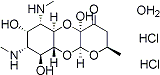Smiles: |O.Cl.Cl.CN[C@@H]3[C@H](O)[C@H](NC)[C@H]2O[C@@]1(O)C(=O)C[C@@H](C)O[C@H]1OC2[C@H]3O|
 Catalog No.: 736S834 Product Name: Spectinomycin dihydrochloride hydrate CAS Number: 21736-83-4 MDL Number: MFCD03788015 Formula: C14H28Cl2N2O8 FW: MSDS PricingSmiles: |Cl.CN[C@@H]3[C@H](O)[C@H](NC)[C@H]2O[C@@]1(O)C(=O)C[C@@H](C)O[C@H]1OC2[C@H]3O|
 Catalog No.: 695S778 Product Name: Spectinomycin HCl CAS Number: 1695-77-8 MDL Number: MFCD06662299 Formula: C14H25ClN2O7 FW: MSDS Pricing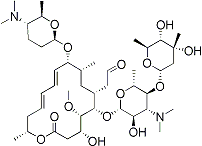Smiles: |CO[C@H]2[C@H](O)CC(=O)O[C@H](C)C/C=C/C=C/[C@H](O[C@H]1CC[C@H](N(C)C)[C@@H](C)O1)[C@H](C)C[C@H](CC=O)[C@@H]2O[C@@H]4O[C@H](C)[C@@H](O[C@H]3C[C@@](C)(O)[C@@H](O)[C@H](C)O3)[C@H](N(C)C)[C@H]4O|
 Catalog No.: 025S818 Product Name: Spiramycin i CAS Number: 8025-81-8 MDL Number: MFCD00242797 Formula: C43H74N2O14 FW: MSDS PricingSmiles: |CN[C@H]1[C@H](O)[C@@H](O)[C@H](CO)O[C@H]1O[C@H]3[C@H](O[C@H]2[C@H](O)[C@@H](O)[C@H](NC(=N)N)[C@@H](O)[C@@H]2NC(=N)N)O[C@@H](C)[C@]3(O)C=O|
 Catalog No.: 057S921 Product Name: Streptomycin CAS Number: 57-92-1 MDL Number: MFCD00072108 Formula: C21H39N7O12 FW: MSDS Pricing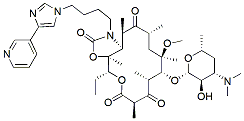Smiles: |CC[C@H]4OC(=O)[C@H](C)C(=O)[C@H](C)[C@@H](O[C@@H]1O[C@H](C)C[C@H](N(C)C)[C@H]1O)[C@](C)(OC)C[C@@H](C)C(=O)[C@H](C)[C@@H]5N(CCCCn3cnc(c2cccnc2)c3)C(=O)O[C@]45C|
 Catalog No.: 114T484 Product Name: Telithromycin CAS Number: 191114-48-4 MDL Number: MFCD04117983 Formula: C43H65N5O10 FW: MSDS Pricing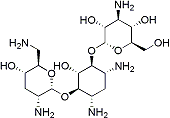Smiles: |NC[C@H]3O[C@H](O[C@@H]2[C@@H](N)C[C@@H](N)[C@H](O[C@H]1O[C@H](CO)[C@@H](O)[C@H](N)[C@H]1O)[C@H]2O)[C@H](N)C[C@@H]3O|
 Catalog No.: 986T564 Product Name: Tobramycin CAS Number: 32986-56-4 MDL Number: MFCD00077885 Formula: C18H37N5O9 FW: MSDS PricingSmiles: |O=S(=O)(O)O.NC[C@H]3O[C@H](O[C@@H]2[C@@H](N)C[C@@H](N)[C@H](O[C@H]1O[C@H](CO)[C@@H](O)[C@H](N)[C@H]1O)[C@H]2O)[C@H](N)C[C@@H]3O|
 Catalog No.: 842T071 Product Name: Tobramycin sulfate CAS Number: 49842-07-1 MDL Number: MFCD00133864 Formula: C18H39N5O13S FW: MSDS PricingSmiles: O[C@H]1[C@@H](O[C@@H]2[C@@H](O)[C@H](O[C@@H]3[C@H](N)C[C@H](O)[C@@H](CN)O3)[C@@H](N)C[C@H]2N)O[C@H](CO)[C@@H](O)[C@@H]1N.O[C@H]4[C@@H](O[C@@H]5[C@@H](O)[C@H](O[C@@H]6[C@H](N)C[C@H](O)[C@@H](CN)O6)[C@@H](N)C[C@H]5N)O[C@H](CO)[C@@H](O)[C@@H]4N.O=S(O)(O)=O.O=S(O)(O)=O.O=S(O)(O)=O.O=S(O)(O)=O.O=S(O)(O)=O
 Catalog No.: 645T275 Product Name: Tobramycin sulfate CAS Number: 79645-27-5 MDL Number: Formula: C36H84N10O38S5 FW: MSDS Pricing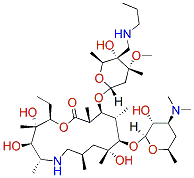Smiles: |CCCNC[C@]3(O)[C@H](C)O[C@@H](O[C@@H]2C(C)C(=O)O[C@H](CC)[C@@](C)(O)[C@H](O)[C@@H](C)NC[C@H](C)C[C@@](C)(O)[C@H](O[C@@H]1O[C@H](C)C[C@H](N(C)C)[C@H]1O)[C@H]2C)C[C@@]3(C)OC|
 Catalog No.: 500T964 Product Name: Tulathromycin A CAS Number: 217500-96-4 MDL Number: MFCD09838804 Formula: C41H79N3O12 FW: MSDS Pricing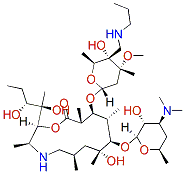Smiles: |CCCNC[C@]3(O)[C@H](C)O[C@@H](O[C@@H]2[C@@H](C)C(=O)O[C@H]([C@](C)(O)[C@H](O)CC)[C@H](C)NC[C@H](C)C[C@@](C)(O)[C@H](O[C@@H]1O[C@H](C)C[C@H](N(C)C)[C@H]1O)[C@H]2C)C[C@@]3(C)OC|
 Catalog No.: 755T126 Product Name: Tulathromycin b CAS Number: 280755-12-6 MDL Number: MFCD09838805 Formula: C41H79N3O12 FW: MSDS Pricing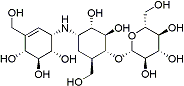Smiles: |OC/C3=C/[C@H](N[C@H]2C[C@H](CO)[C@@H](O[C@@H]1O[C@H](CO)[C@@H](O)[C@H](O)[C@H]1O)[C@H](O)[C@H]2O)[C@H](O)[C@@H](O)[C@@H]3O|
 Catalog No.: 248V478 Product Name: Validamycin CAS Number: 37248-47-8 MDL Number: MFCD01718201 Formula: C20H35NO13 FW: MSDS Pricing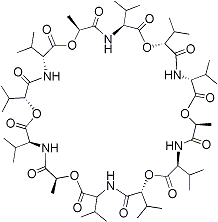Smiles: |CC(C)[C@@H]1NC(=O)[C@H](C)OC(=O)[C@@H](C(C)C)NC(=O)[C@@H](C(C)C)OC(=O)[C@H](C(C)C)NC(=O)[C@H](C)OC(=O)[C@@H](C(C)C)NC(=O)[C@@H](C(C)C)OC(=O)[C@H](C(C)C)NC(=O)[C@H](C)OC(=O)C(C(C)C)NC(=O)[C@@H](C(C)C)OC1=O|
 Catalog No.: 001V958 Product Name: Valinomycin CAS Number: 2001-95-8 MDL Number: MFCD00005114 Formula: C54H90N6O18 FW: MSDS PricingSmiles: |CN[C@H](CC(C)C)C(=O)N[C@H]6C(=O)N[C@@H](CC(N)=O)C(=O)N[C@H]4C(=O)N[C@H]2C(=O)N[C@H](C(=O)N[C@H](C(=O)O)c1cc(O)cc(O)c1c3cc2ccc3O)[C@H](O)c%10ccc(Oc7cc4cc(Oc5ccc(cc5Cl)[C@H]6O)c7O[C@@H]8O[C@H](CO)[C@@H](O)[C@H](O)[C@H]8O[C@H]9C[C@](C)(N)[C@H](O)[C@H](C)O9)c(Cl)c%10|
 Catalog No.: 404V906 Product Name: Vancomycin CAS Number: 1404-90-6 MDL Number: MFCD05664587 Formula: C66H75Cl2N9O24 FW: MSDS Pricing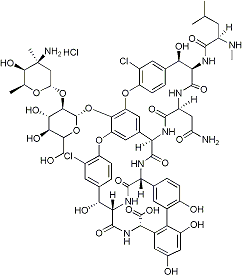Smiles: |Cl.CN[C@H](CC(C)C)C(=O)N[C@H]6C(=O)N[C@@H](CC(N)=O)C(=O)N[C@H]4C(=O)N[C@H]2C(=O)N[C@H](C(=O)N[C@H](C(=O)O)c1cc(O)cc(O)c1c3cc2ccc3O)[C@H](O)c%10ccc(Oc7cc4cc(Oc5ccc(cc5Cl)[C@H]6O)c7O[C@@H]8OC(CO)[C@@H](O)[C@H](O)[C@H]8O[C@H]9C[C@](C)(N)[C@H](O)[C@H](C)O9)c(Cl)c%10|
 Catalog No.: 404V939 Product Name: Vancomycin hydrochloride CAS Number: 1404-93-9 MDL Number: MFCD03613611 Formula: C66H76Cl3N9O24 FW: MSDS Pricing